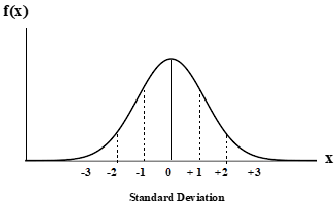# Problem: The average mass of a basketball player on the school team is 185 lbs with a standard deviation of 3.0 lbs. Based on a Gaussian Distribution curve, determine the percentage of basketball players that would weigh below 179 lbs.

###### Problem Details

The average mass of a basketball player on the school team is 185 lbs with a standard deviation of 3.0 lbs. Based on a Gaussian Distribution curve, determine the percentage of basketball players that would weigh below 179 lbs.Frequently Asked Questions

What scientific concept do you need to know in order to solve this problem?

Our tutors have indicated that to solve this problem you will need to apply the The Gaussian Distribution concept. You can view video lessons to learn The Gaussian Distribution. Or if you need more The Gaussian Distribution practice, you can also practice The Gaussian Distribution practice problems.

What is the difficulty of this problem?

Our tutors rated the difficulty ofThe average mass of a basketball player on the school team i...as medium difficulty.

How long does this problem take to solve?

Our expert Analytical Chemistry tutor, Jules took 3 minutes and 2 seconds to solve this problem. You can follow their steps in the video explanation above.